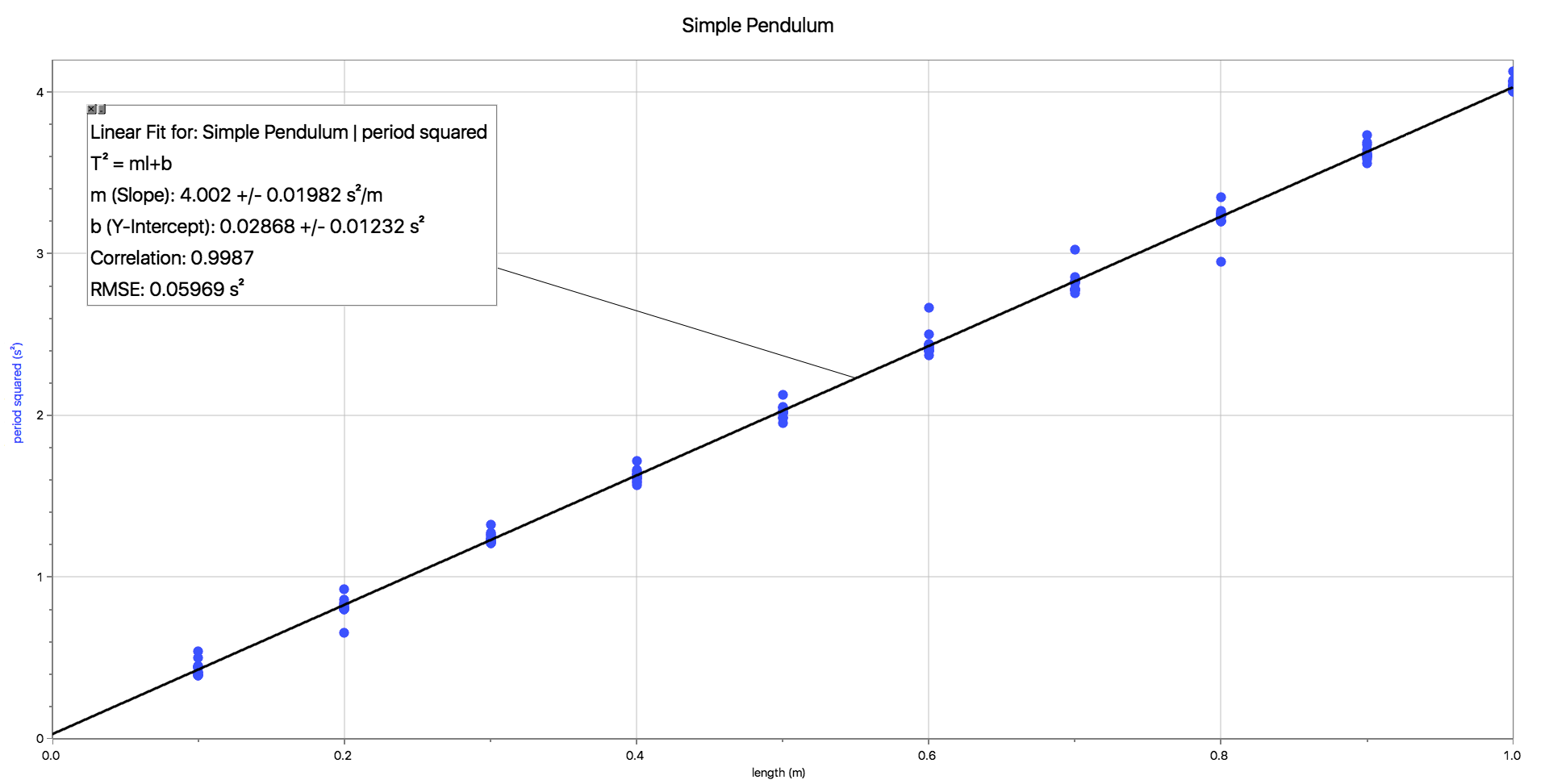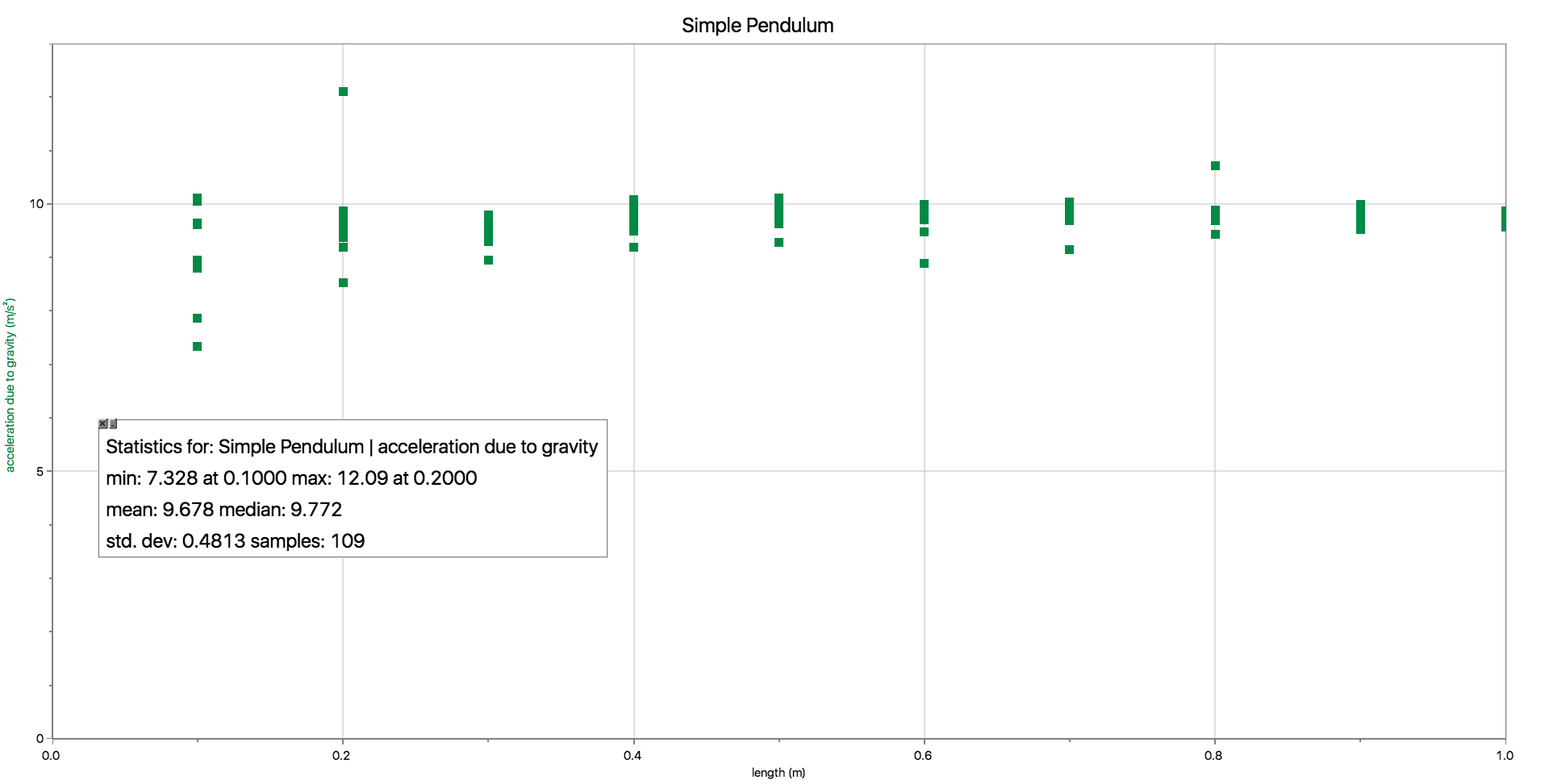The Physics
Hypertextbook
Opus in profectus

# Pendulums

## Practice

### practice problem 1

A "seconds pendulum" has a half period of one second. It takes one second for it to go out (tick) and another second for it to come back (tock).
1. What is the length of a seconds pendulum at a place where gravity equals the standard value of 9.80665 m/s2?
2. What is the period of this same pendulum if it is moved to a location near the equator where gravity equals 9.78 m/s2? How much time does the pendulum lose or gain every 30 days?
3. What is the period of this same pendulum if it is moved to a location near the north pole where gravity equals 9.83 m/s2? How much time does the pendulum lose or gain every 30 days?

#### solution

1. The period of a simple pendulum is described by this equation.

 T = 2π√ ℓ g

Make length the subject.

 ℓ = gT2 4π2

Put numbers in.

 ℓ = (9.80665 m/s2)(2 s)2 4π2

Get answer out. (Keep every digit your calculator gives you. This is a test of precision.)

ℓ = 0.993621386 m

Note how close this is to one meter. In the late 17th century, the the length of a seconds pendulum was proposed as a potential unit definition. Since gravity varies with location, however, this standard could only be set by building a pendulum at a location where gravity was exactly equal to the standard value — something that is effectively impossible.

Let's calculate the number of seconds in 30 days. This part of the question doesn't require it, but we'll need it as a reference for the next two parts.

t = 30×24×60×60 = 2,592,000 s

2. Back to the original equation. Length and gravity are given. Period is the goal.

 T = 2π√ ℓ g

Weaker equatorial gravity in.

 T = 2π√ 0.993621386 m 9.78 m/s2

Slightly longer period out.

T = 2.002723096 s

Half of this is what determines the amount of time lost when this pendulum is used as a time keeping device in its new location.

T½ = 1.001361548 s

Dividing this time into the number of seconds in 30 days gives us the number of seconds counted by our pendulum in its new location.

 n = 2,592,000 s 1.001361548 s

n = 2,588,476 periods

That's a loss of 3524 s every 30 days — nearly an hour (58:44).

3. Repeat.

 T = 2π√ ℓ g

Stronger polar gravity in.

 T = 2π√ 0.993621386 m 9.83 m/s2

Slightly shorter period out.

T = 1.997623207 s

Divide by 2.

T½ = 0.9988116033 s

Divide this into the number of seconds in 30 days.

 n = 2,592,000 s 0.9988116033 s

n = 2,595,084 periods

That's a gain of 3084 s every 30 days — also close to an hour (51:24). Pendulum clocks really need to be designed for a location. Support your local horologist.

### practice problem 2

The Great Clock of Westminster is undoubtedly the world's most famous clock. It is sometimes called "Big Ben", but strictly speaking that is the name of the 13.7 tonne Great Bell. The 12×12×96 meter tower that houses the Great Clock is the iconic symbol of the British Parliament. Many home doorbells and school bells are programmed to play the Westminster Quarters — the four permutations of four notes that announce the quarter hours. During World War II, the Great Clock was a symbol of British resiliency. It continued to operate even after sustaining damage in a German air raid in 1941. (The House of Commons chamber was destroyed in this attack.)
1. The heart of the timekeeping mechanism is a 310 kg, 4.4 m long steel and zinc pendulum. What is the period of the Great Clock's pendulum? This is not a straightforward problem.
1. Begin by calculating the period of a simple pendulum whose length is 4.4 m.
2. The period you just calculated would not be appropriate for a clock of this stature. What is the most sensible value for the period of this pendulum?
3. What is the cause of the discrepancy between your answers to parts i and ii?
2. Pennies are used to regulate the clock mechanism (pre-decimal pennies with the head of Edward VII). Adding one penny causes the clock to gain two-fifths of a second in 24 hours. The pennies are not added to the pendulum bob (it's moving too fast for the pennies to stay on), but are instead placed on a small platform not far from the point of suspension.
1. Read this quote from a 2007 article in the Daily Mail. "When the clock is found to be gaining or losing time, the weight of the pendulum is fractionally adjusted by the addition or removal of an old-fashioned penny piece." While this statement is true, it does not explain how adding a penny affects the operation of the pendulum. What error did the reporter make in his explanation?
2. Why does this method really work; that is, what does adding pennies near the top of the pendulum change about the pendulum?
3. Now for a mathematically difficult question. By what amount did the important characteristic of the pendulum change when a single penny was added near the pivot?

#### solution

1. What is the period of the Great Clock's pendulum?

1. Calculate the period of a simple pendulum whose length is 4.4 m in London where the local gravity is 9.81 m/s2.

 T = 2π√ ℓ g
 T = 2π√ 4.4 m 9.81 m/s2
 T = 4.21 s
2. The period of the Great Clock's pendulum is probably 4 seconds instead of the crazy decimal number we just calculated. That way an engineer could design a counting mechanism such that the hands would cycle a convenient number of times for every rotation — 900 cycles for the minute hand and 10,800 cycles for the hour hand.

 3,600 s 1 cycle = 900 cycles/1 hour 1 h 4 s
 43,200 s 1 cycle = 10,800 cycles/12 hours 12 h 4 s
3. In part a i we assumed the pendulum was a simple pendulum — one with all the mass concentrated at a point connected to its pivot by a massless, inextensible string. In part a ii we assumed the pendulum would be used in a working clock — one designed to match the cultural definitions of a second, minute, hour, and day. The reason for the discrepancy is that the pendulum of the Great Clock is a physical pendulum. Instead of an infinitesimally small mass at the end, there's a finite (but concentrated) lump of material. Instead of a massless string running from the pivot to the mass, there's a massive steel rod that extends a little bit beyond the ideal starting and ending points.

2. How does adding pennies to the pendulum in the Great Clock help to keep it accurate?

1. Although adding pennies to the Great Clock changes its weight (by which we assume the Daily Mail meant its mass) this is not a factor that affects the period of a pendulum (simple or physical). Look at the equation below. Tell me where you see mass.

 T = 2π√ ℓ g
2. Adding pennies to the pendulum of the Great Clock changes its effective length. Since the pennies are added to the top of the platform they shift the center of mass slightly upward. This shortens the effective length of the pendulum. Look at the equation again. Notice how length is one of the symbols. That means length does affect period.

 T = 2π√ ℓ g
3. Now for the mathematically difficult question. You may not have seen this method before. Solve the equation I keep using for length, since that's what the question is about.

 ℓ = gT2 4π2
⇐
 T = 2π√ ℓ g

Perform a propagation of error calculation on the two variables: length () and period (T).

 ∆ℓ = dℓ ∆T dT

It's a calculus thing.

 ∆ℓ = gT ∆T 2π2

Two-fifths of a second in one 24 hour day is the same as 18.5 μs in one 4 s period.

 0.4 s = ∆T 24×60×60 s 4 s

T = 1.85 × 10−5 s = 18.5 μs

Use this number as the uncertainty in the period.

 ∆ℓ = (9.81 m/s2)(4 s) (18.5 μs) 2π2

∆ℓ = 3.68 × 10−5 s = 36.8 μm

Adding pennies to the Great Clock shortens the effective length of its pendulum by about half the width of a human hair.

### practice problem 3

Write something different.

### practice problem 4

simple-pendulum.txt
A classroom full of students performed a simple pendulum experiment. They attached a metal cube to a length of string and let it swing freely from a horizontal clamp. They recorded the length and the period for pendulums with ten convenient lengths. Use these results to determine the acceleration due to gravity at this location.

#### solution

There are two basic approaches to solving this problem graphically — a curve fit or a linear fit. Let's do them in that order.

First method: Start with the equation for the period of a simple pendulum.

 T = 2π√ ℓ g

Compare it to the equation for a generic power curve.

y = Axn

If we let…

 y = T and x = ℓ

then…

 A = 2π/√g and n = ½

Set up a graph of period vs. length and fit the data to a square root curve.Use the constant of proportionality to get the acceleration due to gravity.

g =
 ⎛⎜⎝ 2π ⎞2⎟⎠ A

g =
 ⎛⎜⎝ 2π ⎞2⎟⎠ 2.103

g =  9.742 m/s2

Second method: Square the equation for the period of a simple pendulum.

 T2 = 4π2 ℓ g

Compare it to the equation for a straight line.

y = mx + b

If we let…

 y = T2 and x = ℓ

then…

 m = 4π2/g and b = 0

Set up a graph of period squared vs. length and fit the data to a straight line.Now use the slope to get the acceleration due to gravity.

g =
 4π2 m

g =
 4π2 4.002

g =  9.865 m/s2

Bonus solutions: Start with the equation for the period of a simple pendulum.

 T = 2π√ ℓ g

Solve it for the acceleration due to gravity.

 g = 4π2ℓ T2

Compute g repeatedly, then compute some basic one-variable statistics. This method isn't graphical, but I'm going to display the results on a graph just to be consistent.The most popular choice for the measure of central tendency is probably the mean (g bar).

g = 9.678 m/s2

But the median is also appropriate for this problem (g tilde).

= 9.722 m/s2

How about some rhetorical questions to finish things off?

9.742 m/s2, 9.865 m/s2, 9.678 m/s2, 9.722 m/s2

Which answer is the right answer? All of the methods used were appropriate to the problem and all of the calculations done were error free, so all of them. Which answer is the best answer? That's a question that's best left to a professional statistician. What is the answer supposed to be? The answers we just computed are what they are supposed to be. The problem said to use the numbers given and determine g. We did that. What is the generally accepted value for gravity where the students conducted their experiment? I think it's 9.802 m/s2, but that's not what the problem is about.